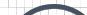Informative Information for the Uninformed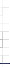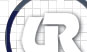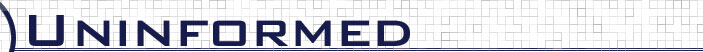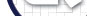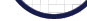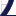Vol 8» 2007.Sep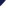# Reachability

The real benefit of the generalizations described in §2 can be realized when attempting to solve a graph reachability problem. By generalizing data flow behaviors to both abstract and concrete generalization tiers, it is possible to reduce the amount of information that must be represented when attempting to determine graph reachability. This is further improved by the fact that data flow paths found at less-specific generalization tiers can be used to progressively qualify potential data flow paths at more-specific generalization tiers. This qualification is possible due to the fact that less-specific data flow paths are associated with more-specific data flow paths at each generalization tier through a one-to-many link table, thus permitting trivial expansion. The benefit of qualifying data flow paths in this fashion is that only the minimal set of information needed to determine reachability must be considered at once at each generalization tier. This can drastically reduce the physical resources required to solve a graph reachability problem by effectively limiting the size of a graph. This general approach is captured by the Progressive Qualified Elaboration (PQE) algorithm described by 3.1. This concept is very similar to the ideas outlined by Schultes' highway hierarchy which is used to optimize fast path discovery when identifying travel routes in road networks.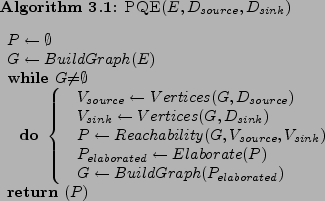For the purposes of this paper, graph reachability is restricted to determining realizable paths between two flow descriptors: a source and a sink. A flow descriptor provides information that is needed to identify corresponding vertices within a graph at each generalization tier. The tables in figure 12 and figure 13 show the information needed to identify source and sink vertices at each generalization tier for the example that will be described in this section.

The PQE algorithm itself requires three parameters. The first parameter,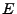, contains the set of generalized elements to be analyzed. For example, it may contain the set of target modules that should be analyzed. The second and third parameters,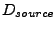and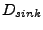, represent the source and sink flow descriptors, respectively.

The first step taken by the algorithm is to define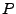as an empty set.will be used to contain the set of reachable paths between an actual set of sources and sinks at a given generalization tier. Afterhas been initialized,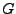is initialized to a flow graph that conveys data flow relationships between the set of elements provided in. The approach taken to construct the flow graph involves retrieving persisted data flow information for the appropriate generalization tier. Onceandhave been initialized, the qualified elaboration process can begin.

For each loop iteration, a check is made to see ifis an empty graph (contains no vertices). Ifis empty, the loop terminates. Ifis not an empty graph, reachable paths between the actual set of sources and sinks are determined. This is accomplished by first identifying the vertices inthat are associated with the flow descriptorsandat the current generalization tier. The actual set of sources and sinks found to be associated with these descriptors are stored in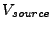and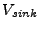, respectively. With the set of actual source and sink vertices identified, a reachability algorithm,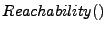, can be used to determine the set of reachable paths in flow graphbetween the two sets of vertices,and. The result of this determination is stored in. The final step in the iteration involves using qualified elaboration to construct a new flow graph containing more-specific data flow paths which are qualified by the set of data flow paths encountered in the reachable paths found in. This set is then elaborated to a subset that contains the associated data flow paths from the next, more specific tier, such as by elaborating to a subset of basic blocks data flow paths from a more general set of procedure data flow paths. The result of the elaboration is stored in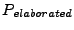. Finally, a new flow graph is constructed and stored inusing the elaborated set of flow paths contained within.

When it is not possible to obtain a more-detailed flow set, such as when the instruction tier is reached, an empty graph is created and the algorithm completes by returning. In the final iteration,contains the most detailed set of reachable data flow paths found between the source and sink flow descriptors. The benefit of approaching graph reachability problems in this fashion is that only a subset of the elements at any generalization tier need to be considered at once. These subsets are dictated by the set of reachable data flow paths found at each preceding generalization tier. In this manner, the subset of procedure data flow paths that need to be considered would be effectively qualified by the set of data types and modules found to be involved in data flow paths between the source and sink flow descriptors at less-specific tiers.

For the purposes of this paper, thealgorithm is designed to consider realizable paths at each generalization tier in manner that is similar to the concept described by Reps et al. This involves traversing the graph in context-sensitive fashion. To accomplish this, the algorithm keeps a scope stack at each generalization tier. The scope may be an assembly, a type, or a procedure. When data is passed through to a formal input parameter, the scope for the formal input parameter is pushed onto the stack. When data is returned through a formal output parameter to another location, the algorithm ensures that the scope that is being returned to is the parent scope. In this way, only realizable paths are considered at each generalization tier which limits the number of paths that must be considered and also has the benefit of producing more accurate results.

The specific algorithm used for the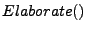function involves using the set of data flow paths found at a less-specific tier to identify the set of more-specific data flow paths that have been generalized. This is accomplished by simply using the one-to-many link tables that were populated during generalization to determine the subset of data flow paths that must be considered at the next generalization tier. For example, elaborating from a set of procedure data flow paths would involve determining the complete set of basic block data flow paths that have been generalized by the affected set of procedure data flow paths.

Based on this general description of the algorithm, it is useful to consider a concrete example This section provides a concrete illustration by determining reachability between a source and a sink using an example web application that consists of a web client and a web service component. This is illustrated by progressively drilling down through each generalization tier starting from the least-specific tier, the abstract tier, and working toward the most-specific tier, the instruction tier. At each tier, a description of the number of data flow paths that must be represented and the number of reachable data flow paths found is given. This particular example will attempt to determine concrete reachable data flow paths between the return value of HttpRequest.get_QueryString and the first formal input parameter of Process.Start. The implications of a reachable path between these two points could be indicative of a command injection vulnerability within the application. The tables in figure 12 and figure 13 show the flow descriptors for the source and sink, respectively. These flow descriptors are used to identify associated vertices at each generalization tier.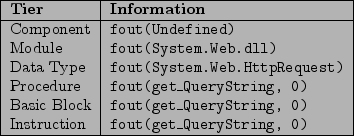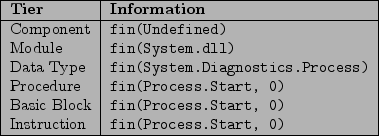For this illustration, there is in fact a data flow path that exists from the source descriptor to the sink descriptor. However, unlike conventional data flow paths, this data flow path happens to cross an abstract boundary between the two components. In this case, data is passed from the web client component through an HTTP request to a method hosted by the web service component. This path can be seen by first looking at a portion of the web client code:

class Program {
static void Main(string[] args) {
HttpRequest request = new HttpRequest(
"a","b","c");
WebClient client = new WebClient();

client.ExecuteCommand(
request.QueryString["abc"]);
}
}
[WebServiceBinding]
public class WebClient : SoapHttpClientProtocol {
[SoapDocumentMethod]
public void ExecuteCommand(string command) {
Invoke("ExecuteCommand",
new object[] { command });
}
}


In this contrived example, data is shown as being passed from a query string obtained from what is presumably a real HTTP request to the client portion of the web service method ExecuteCommand. The web service application, in turn, contains the following code:

[WebService]
public class WebService {
[WebMethod]
public void ExecuteCommand(string command) {
System.Diagnostics.Process.Start(command);
}
}


In conventional tools, it would not be possible to directly model this data flow path because the data flow path is indirect. However, using a simple methodology to bridge the client-side formal input parameters with the server-side formal input parameters at the instruction tier, it is possible to connect the two and represent data flow between the two conceptual software elements at each generalization tier. The following sections will provide visual examples of how the PQE algorithm narrows down and eliminates unnecessary data flow paths at each generalization tier by progressively qualifying data flow information. One thing to note about the graphs at each tier is that implicit edges have been created between formal input and output parameters that reside in external (un-analyzed) libraries. This is done under the assumption that a formal input parameter may affect a formal output parameter in some way in the context of the code that is not analyzed. If all target code paths have been analyzed, then this is not necessary. The graphs shown at each tier were automatically generated but have been modified to allow them to fit within the margins of this document and in some cases highlight important features.

Subsections